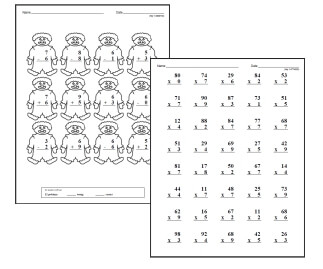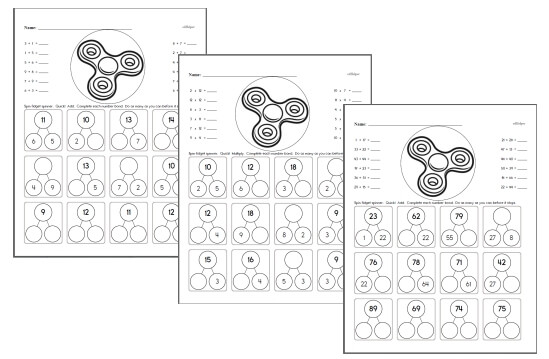Worksheets and No Prep Teaching Resources Math WorksheetsMath Facts Addition, Subtraction, Multiplication, and Division

Math Facts with Fidget SpinnersWorkbooks to practice math facts using fidget spinners

Math Facts with Pictures

Math Facts

Math Facts with Scoring Box at Bottom

Addition Facts: Numbers 0 to 9
Addition Facts: Numbers 0 to 9
Large Fonts (fewer problems)
Small Fonts (more problems)

Addition Facts: Numbers 0 to 9 - Cross out the incorrect facts
Addition Facts: Numbers 0 to 9 - Cross out the incorrect facts
Large Fonts (fewer problems)
Small Fonts (more problems)

Addition Facts: Focusing on a number

Quick Subtraction Math Facts

Subtraction Facts: Numbers 0 to 9
Subtraction Facts: Numbers 0 to 9
Large Fonts (fewer problems)
Small Fonts (more problems)

Subtraction Facts: Numbers 0 to 9 - Cross out the incorrect facts
Subtraction Facts: Numbers 0 to 9 - Cross out the incorrect facts
Large Fonts (fewer problems)
Small Fonts (more problems)

Subtraction Facts: Focusing on a number
Subtraction with the number 1
Subtraction with the number 2
Subtraction with the number 3
Subtraction with the number 4
Subtraction with the number 5
Subtraction with the number 6
Subtraction with the number 7
Subtraction with the number 8
Subtraction with the number 9

2-Digit Subtraction Facts
2-Digit subtraction
2-Digit subtraction with no regrouping
2-Digit subtraction with all regrouping

Super Silly Squares

Subtraction Squares

Multiplication Squares

Mixed Math Square Puzzles

Have a suggestion or would like to leave feedback?